In this post, I show a trick to do moving average calculation (can be extended to other operations requiring windowing functions) that is super fast.

Often, SAS analysts need to conduct moving average calculation and there are several options by the order of preference:

1. PROC EXPAND
2. DATA STEP
3. PROC SQL

But many sites may not licensed SAS/ETS to use PROC EXPAND and doing moving average in DATA STEP requires some coding and is error prone. PROC SQL is a natural choice for junior programmers and in many business cases the only solution, but SAS's PROC SQL lacks windowing functions that are available in many DBs to facilitate moving average calculation. One technique people usually use is CROSS JOIN, which is very expensive and not a viable solution for even medium sized data set. In this post, I show a trick to do moving average calculation (can be extended to other operations requiring windowing functions) that is super fast.

Consider the simplest moving average calculation where the trailing K observations are included in the calculation, namely MA(K), here we set K=5. We first generate a 20 obs sample data, where variable ID is to be used for windowing and the variable X is to be used in MA calculation, and then we apply the standard CROSS JOIN to first examine the resulting data, Non-Grouped, just to understand how to leverage the data structure.
``%let nobs=20;%let ndiff=-5;data list;     do id=1 to &nobs;     x=id*2;  output;  end;run;options notes;options fullstimer;proc sql;      create table ma as   select a.id as aid, b.id as bid, a.id-b.id as diff, a.x as ax, b.x as bx   from   list as a, list as b   where a.id>=b.id and (a.id-b.id)<= abs(&ndiff)-1         having aid-bid>=(&ndiff+1)   order by aid, bid   ;quit;``

From the resulting data set, it is hard to find a clue, now let's sort by "bid" column in this data set:
﻿
From this sorted data, it is clear that we actually don't have to CROSS JOIN the whole original data set, but instead, we can generate an "operation" data set that contains the difference value, and let the original data set CROSS JOIN with this much smaller "operation" data set, and all the data we need to use for MA calculation will be there. Now let's do it: CROSS JOIN original data with "operation" data, sort by (a.id+ops), which is actually "bid' in sorted data set;
``%let ndiff=5;data operation;     do ops = 0 to &ndiff by 1;     weight=1;     output;  end;run;proc sql;      create table ma2 as   select a.id as aid, b.ops, a.id+b.ops as id2, a.x*b.weight as ax      from   list as a, operation as b       order by id2, aid      ;quit;``
Note that in above code, it is necessary to have a.x multiply by b.weight so that the data can be inter-leaved, otherwise the same X value from original table will be output and MA calculation will be failed. The explicit weight variable actually adds in more flexibility to the whole MA calculation. While setting it to be 1 for all obs result in a simple MA calculation, assign different weights will help to resolve more complex MA computing, such as giving further observations less weight for a decayed MA. If different K parameter in MA(K) calculations are required, only the operation data set need to be updated which is trivial job. Now the actual code template for MA(K) calculation will be:
``%let ndiff=5;data operation;     do ops = 0 to &ndiff by 1;     weight=1;     output;  end;run;proc sql noprint;      select max(id) into :maxid   from   list;quit;proc sql;      create table ma2 as   select a.id+b.ops as id2, avg(a.x*b.weight) as MA      from   list as a, operation as b       group by id2    having id2>=&ndiff     and  id2<=&maxid   order by id2        ;quit;``
With this new method, it is interesting to compare it to the expensive self CROSS JOIN as well as to PROC EXPAND. On my workstation (Intel i5 3.8Ghz, 32GB memory, 1TB 72K HDD), self CROSS JOIN is prohibitively long in running time (if data is large) while the new method uses only 2X as much time as PROC EXPAND, both time consumptions are trivial comparing to self CROSS JOIN. Time consumption shown below is in "second".

Below is the code readers can run and compare yourselves.
``%macro test(nobs, ndiff);options nonotes;data list;     do id=1 to &nobs;     x=id*2;  output;  end;run;%let t0 = %sysfunc(time());options fullstimer;proc sql;      create table ma as   select a.id, avg(b.x) as ma   from   list as a, list as b   where a.id>=b.id and (a.id-b.id)<= &ndiff-1   group by a.id   having id>=abs(&ndiff)   ;quit;%let t1 = %sysfunc(time());proc expand data=list out=ma2 method=none;     convert x=ma / transformout=(movave 5);run;%let t2 = %sysfunc(time());%let ndiff=5;data operation;     do ops = 0 to &ndiff-1 by 1;     weight=1;     output;  end;run;proc sql noprint;      select max(id) into :maxid   from   list;quit;proc sql;      create table ma3 as   select a.id+b.ops as id2, avg(a.x*b.weight) as ma   from   list as a, operation as b   group by id2         having id2>=abs(&ndiff)      ;quit;%let t3 = %sysfunc(time());%let d1 = %sysfunc(round( %sysevalf(&t1 - &t0), 0.001));%let d2 = %sysfunc(round( %sysevalf(&t2 - &t1), 0.001));%let d3 = %sysfunc(round( %sysevalf(&t3 - &t2), 0.001));%put SelfXJoin : &d1, EXPAND: &d2, Operation: &d3;options notes;%mend;dm log 'clear';%test(5000, -40);%test(10000, -40);%test(15000, -40);%test(20000, -40);%test(25000, -40);%test(30000, -40);``Like millions of other Americans, I recently was asked to make a decision of tremendous importance to my household -- a decision that would affect the welfare of everyone in my family. That decision, of course, was whether to continue to receive Netflix movies by mail, or opt for the less-expensive "streaming only" subscription.

Let me just say this up front: we love our Netflix subscription.  We subscribed way back in 2005 on the low-cost "one-disc-at-a-time" plan, and since then we've seen over 180 movies that we received on-loan, delivered via the US Postal Service.  Many of these were movies that we never would have seen otherwise: older films, independent films, and many other titles that would have been difficult to find at a local video rental shop.

But having arrived at this crossroads, it's a good time to try and measure just how much the DVD-by-mail option would cost us, and then we can decide what action to take.  And of course, I used SAS Enterprise Guide to analyze my Netflix history, and thus created additional insight about the movie-rental pattern of my household.

## Getting my account history into SAS

One of the things that I like about Netflix is how they allow you to see your entire account history online.   At the time that I'm writing this, this URL will get you to your complete DVD shipping activity (although this could change, as Netflix is restructuring their DVD-by-mail business):

https://www.netflix.com/RentalActivity?all=true

In order for that URL to work, you must be already signed in to your Netflix account in your web browser.  While there are several ways to turn this web page into data, I found the easiest method within Microsoft Excel.  On the Data ribbon menu, select Get External Data->From Web.  On the New Web Query window, paste the URL in the Address field and click Go.  You'll see a preview of the web page where you can select the table of your account history to import as data.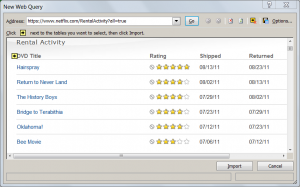When the content is transferred into the Excel spreadsheet, I saved the file (as NetflixHistory.xlsx), and closed Microsoft Excel.  The spreadsheet doesn't look like data that's ready to analyze yet (lots of extra rows and space as you can see in the example below), but that's okay.  I can fix all of that easily in SAS.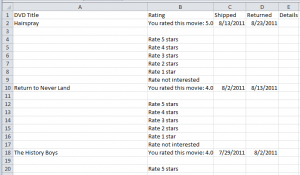With the data now in an Excel spreadsheet, I fired up SAS Enterprise Guide and selected File->Import Data. After just a few clicks through the wizard, I've got the data in a work data set.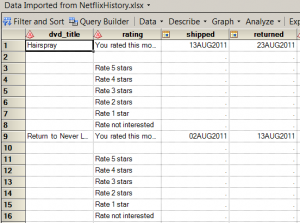## Cleaning the data and calculating the value

The data records for the account history are very simple, containing just four fields for each movie: DVD_Title, Rating (whether we liked it), Shipped (date when Netflix shipped the movie out to me), and Returned (date when Netflix received the movie back from me).  My goal for this project is to measure value, and there are no measures in this data...yet.  I also need to filter out the "garbage" rows -- those values that had some purpose in the HTML page, but don't add anything to my analysis.

I'm going to accomplish all of this within the SAS Enterprise Guide query builder, building it all into a single step.  First, I need to design a few filters to clean up the data, as shown here: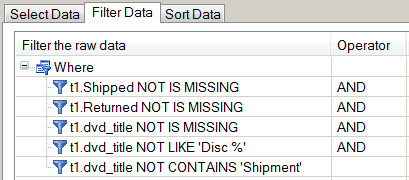The first three filters will drop all of the rows that don't contain information about a DVD title or shipment.  The last two filters will drop any records that reflect multi-disc shipments, or the occasional replacement shipment from when I reported a damaged disc.  Those are rare events, and they don't contain any information that I need to include in my analysis.

Next, I want to calculate some measures.  The most obvious measure to calculate is "How many days did we have the movie" -- the difference between the Shipped Date and Received Date.  And while that number will be interesting, by itself it doesn't convey value or cost.  I want a number that I can express in dollar terms.  To come up with that number, I will employ the tried-and-true method used by data hackers all over the world: I will Make Something Up.

In this case, I'm going to create a formula that reflects my cost for each movie.  That formula is:

(Netflix Monthly Fee / Days In a Month) * Days We Had the Movie = Cost of the Movie

Using the query builder, I calculated new columns with these values.  I assumed the fee was \$10/month (varied over time, but this constant is good enough) and that there are 30 days in a month (again, a "good enough" constant).  Here are the new columns in the query builder: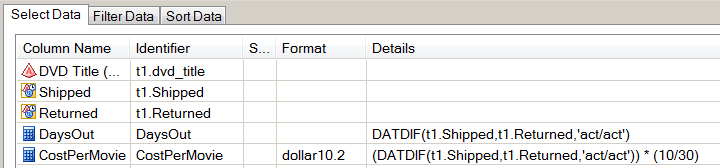After applying these filters and calculations, I finally have a result set that looks interesting to analyze: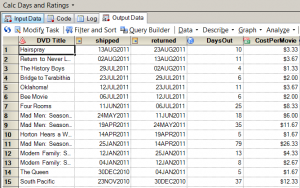By sorting this data by CostPerMovie, I can see that the "cheapest movies" were those that we had out for only 3 days, which is the fastest possible turnaround (example: receive in the mailbox on Monday, watch Monday night, mail out on Tuesday, Netflix receives on Wednesday and ships the next DVD in our queue).  By my reckoning, those DVDs cost just \$1 to watch.  The most expensive movie in my list came to \$26.33, a Mad Men DVD that sat for 79 days while we obviously had other things to do besides watch movies.

## Visualizing the results

To visualize the "Days Out" as a time series, I used the SGSCATTER procedure to generate a simple plot.  You can see that at the start of our Netflix subscription, we were enthusiastic about watching the movies immediately after we received them, and then returning them in order to release the next title from our queue.  These are where the DaysOut values are closer to zero.  But as time goes on and Life Gets Busy, there are more occurrences of "extended-period loans", with higher values for DaysOut.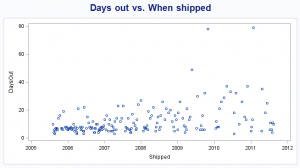Because I've calculated the cost/movie with my sophisticated model, I can plot the cost over time by using the SERIES statement in PROC SGPLOT, with this result: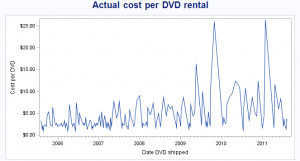This plot makes it easy to see that I've had a few "high cost" DVDs.  But it's still difficult to determine an actual trend from this, because the plot is -- and this is a technical term -- "too jumpy".  To remedy that, I used another task in SAS Enterprise Guide -- one that I probably have no business using because I don't fully understand it.  I used the Prepare Time Series Data task (found under the Tasks->Time Series menu) to accomplish two things:

• Calculate the moving average of the CostPerMovie over each 10-movie interval, in an effort to "smooth out" the variance among these values.
• Interpolate the CostPerMovie value for all dates that are covered by these data, so that on any given day I can see the "going rate" of my CostPerMovie, even if that date is not a Shipped Date or Received Date.

This magic happens behind the scenes by using PROC EXPAND, part of SAS/ETS.  And although PROC EXPAND creates some nice plots by using ODS statistical graphics, I created my own series plot again by using PROC SGPLOT: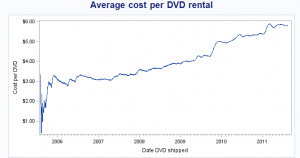This plot confirms what I already know: our movies have become more expensive over the past 6 years of my subscription.  But more importantly, it tells me by how much: from an initial cost of \$3-4, it's now up to nearly \$6 per movie -- based solely on our pattern of use.

Important note: The data I collected and analyzed covers only the DVDs we've had shipped to us.  It does not include any movies or shows that we've watched by streaming the content over the Internet.  The "instant watch" feature is an important component of the Netflix model, and we do use this quite a bit.  I know that this accounts for much of the decrease in frequency for our DVD watching.  But by changing their pricing model, Netflix basically asked the question: how much is it worth to you to continue receiving movies by mail, independent of the streaming content?

And I answered that question: it's not worth \$6 per DVD to me (as I reckon it, given my pattern of use).  Like millions of others, I've opted out of the DVD-by-mail service.  But we've kept the streaming service!  In a future post, I'll take a look at how we use the streaming content and what value we receive from it. [UPDATE: Here it is, the analysis of my streaming account.]We demonstrate a comparison among various implementations of Rolling Regression in SAS and show that the fastest implementation is over 3200X faster than traditional BY-processing approach.

More often than not, we encounter a problem where an OLS over a rolling time window is required, see , , , , , , , for a few examples.

One solution is to resort to SAS MACRO, but it is extremely inefficient and can't handle large dataset in reality, . This method is shown below as Method. It couldn't finish the test in allowable time using the sample data below.

The other common solution is to use the BY-processing capability of PROC REG after re-shaping the data into appropriate format, see , . This method is demonstrated as Method below. While certainly much better than above one, it is still not the fastest and requires more memory.

The third solution comes to play by recognizing that in OLS, what you need is the SSCP and you can easily build up the SSCP over rolling time window by resorting to PROC EXPAND. This is demonstrated as Method below. This approach will further improve the speed but still requires large amount of memory if the data is big and many rolling windows are generated.

Since what we need to do is to build the SSCP matrix and obtain the coefficient estimates based on the informaiton in SSCP, we can certainly code this in a DATA Step using ADJUST operator, which provides a solution that is both fast and low memory occupancy. See  for an introduction to ADJUST operator. To make this even faster, a modification of ADJUST operator, the SWEEP operator, can be used. For an introduction to SWEEP operator, see , . In the code below, Method implements the ADJUST operator, while Method implements the SWEEP operator.

The experiment literally runs 499980 regressions each with 20 observations and 2 predictors, and the results are shown below:

Real Time    |        CPU Time          | Memory
=====================================================

Method 0 |    1.01 (seconds)   |    1.01 (seconds)        |    611K

Method 1 |    0.25 (seconds)   |    0.24 (seconds)        |    432K

Method 2 |    1.61 (seconds)   |    0.94 (seconds)        | 50381K

Method 3 |  80.54 (seconds)   |   79.61 (seconds)       |  2322K

Method 4 |         Failed           |           Failed               |    Failed
=====================================================

Reference:
MYSAS.NET, http://www.mysas.net/forum/viewtopic.php?f=4&t=8070
MYSAS.NET, http://www.mysas.net/forum/viewtopic.php?f=4&t=7898
SAS-L, http://www.listserv.uga.edu/cgi-bin/wa?A2=ind0604D&L=sas-l&P=R32485
SAS-L, http://www.listserv.uga.edu/cgi-bin/wa?A2=ind0704C&L=sas-l&P=R3305
SAS-L, http://www.listserv.uga.edu/cgi-bin/wa?A2=ind0802C&L=sas-l&P=R9746
SAS-L, http://www.listserv.uga.edu/cgi-bin/wa?A2=ind0801C&L=sas-l&P=R14671
SAS-L, http://www.listserv.uga.edu/cgi-bin/wa?A2=ind0810A&L=sas-l&P=R19135
SAS-L, http://www.listserv.uga.edu/cgi-bin/wa?A2=ind0802C&L=sas-l&P=R13489
Michael D Boldin, "Programming Rolling Regressions in SAS", Proceedings of NESUG, 2007
SAS-L, http://www.listserv.uga.edu/cgi-bin/wa?A2=ind0604D&L=sas-l&D=0&P=56926
J. H. Goodnight, "The Sweep Operator: Its Importance in Statistical Computing", SAS Tech Report R-106, 1978
Kenneth Lange, "Numerical Analysis for Statisticians", Springer, 1998

``proc datasets library=work kill; run;options fullstimer;data test;     do seq=1 to 500000;          x1=rannor(9347957);          *x2=rannor(876769)+0.1*x1;          epsilon=rannor(938647)*0.5;          y = 1.5 + 0.5*x1 +epsilon;          output;     end;run;/* Method 0.*/sasfile test load;data res0;        set test;  array _x{3,3} _temporary_ ;  array _a{3,3} _temporary_ ;  array _tempval{5, 20} _temporary_ ;  m=mod(_n_-1, 20)+1;  _tempval[1, m]=x1;   _tempval[2, m]=y;  _tempval[3, m]=x1**2;  _tempval[4, m]=x1*y;  _tempval[5, m]=y**2;  link filler;  if _n_>=20 then do;        if _n_>20 then do;                    m2=mod(_n_-20, 20)+1;       _x[1,2]+(-_tempval[1, m2]);       _x[1,3]+(-_tempval[2, m2]);       _x[2,2]+(-_tempval[3, m2]);       _x[2,3]+(-_tempval[4, m2]);       _x[3,3]+(-_tempval[5, m2]);     end;        do i=1 to dim(_a, 1);         do j=1 to dim(_a, 2);          _a[i, j]=_x[i, j];      end;     end;                          do k=1 to dim(_a, 1)-1;         link adjust;              end;     Intercept=_a[1,3]; beta=_a[2,3];     keep seq   intercept  beta;     output;  end;  return;filler:   _x[1,1]=20; _x[1,2]+x1; _x[1,3]+y;   _x[2,2]+_tempval[3,m];  _x[2,3]+_tempval[4,m]; _x[3,3]+_tempval[5,m];   _x[2,1]=_x[1,2]; _x[3,1]=_x[1,3]; _x[3,2]=_x[2,3]; return;adjust:    B=_a[k, k]; do j=1 to dim(_a, 2);     _a[k, j]=_a[k, j]/B; end; do i=1 to dim(_a, 1);     if i ^=k then do;          B=_a[i, k];    do j=1 to dim(_a, 2);        _a[i, j]=_a[i, j]-B*_a[k, j];    end;  end; end;return;run;sasfile test close;/* Method 1.*/sasfile test load;data rest0;        set test;  array _x{4} _temporary_;  array _a{2,20}  _temporary_;  m=mod(_n_-1, 20)+1;  _a[1, m]=x1; _a[2,m]=y;  link filler;  m2=mod(_n_-20, 20)+1;  if _n_>=20 then do;    if _n_>20 then do;              link deduct;    end;    beta=(_x-_x*_x/20)/(_x-_x**2/20);    intercept=_x/20 - beta*_x/20;    keep  seq   intercept  beta  ;    output;  end;  return;       filler:     _x+x1;  _x+x1*y;  _x+x1**2;  _x+y;return;deduct:     _x=_x-_a[1,m2];   _x=_x-_a[1,m2]*_a[2,m2];  _x=_x-_a[1,m2]**2;  _x=_x-_a[2,m2];return;run;sasfile test close;/* Method 2.*/%macro wrap;%let window=20;%let diff=%eval(&window-0);data testv/view=testv;     set test;       xy=x1*y;  run;proc expand data=testv  method=none  out=summary(keep=seq sumxy  sumx1  sumy  ussx1  may  max);       convert  x1=sumx1/transformout=(movsum &diff);       convert  xy=sumxy/transformout=(movsum &diff);       convert  x1=ussx1/transformout=(movuss &diff);       convert  y =sumy /transformout=(movsum &diff);       convert  y =may / transformout=(movave &diff);       convert  x1 =max / transformout=(movave &diff);  run;data result1;     set summary(firstobs=&window);       beta = (sumxy - sumx1*sumy/&window)/(ussx1 - sumx1/&window.*sumx1);         alpha= may - beta*max;       keep seq  beta  alpha; run;%mend;%let t0=%sysfunc(datetime(), datetime24.);*options nosource nonotes;%wrap;options source notes;%let t1=%sysfunc(datetime(), datetime24.);%put Start @ &t0;%put End   @ &t1; /* Method 3.*/%let t0=%sysfunc(datetime(), datetime.); data test2v/view=test2v;       set test;       array _x{2, 20} _temporary_ (20*0 20*0);       k=mod(_n_-1, 20)+1;       _x[1, k]=x1; _x[2, k]=y;       if _n_>=20 then do;          do j=1 to dim(_x, 2);               x=_x[1, j]; y=_x[2, j];               output;               keep seq x y;            end;       end;run;ods select none;proc  reg data=test2v  outest=res2(keep=seq x intercept);         by seq;         model y = x;run;quit;ods select all;%let t1=%sysfunc(datetime(), datetime.);%put Start @ &t0;%put End   @ &t1;/* Method 4. */%macro wrap;options nonotes;ods select none;%do i=20 %to 500000;       %let fo=%eval(&i-19);       proc reg data=test(firstobs=&fo  obs=&i)  outest=_xres(keep=x1 intercept);           model y =x1;       run;quit;      %if %eval(&i=20) %then %do;          data res3; set _xres; run;      %end;      %else %do;        proc append base=res3  data=_xres; run;      %end;%end;ods select all;data res3;       set res3;       time=19+_n_;run;options notes;%mend;%let t0=%sysfunc(datetime(), datetime.);%wrap;%let t1=%sysfunc(datetime(), datetime.);%put Start @ &t0;%put End   @ &t1;``